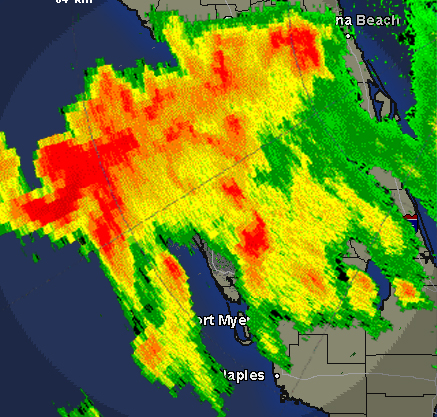# Building a Rain Gauge, part 2

Back to part 1

### What Does a Rain Gauge Really Tell Us?

Now that we know the proper shape and location for a rain gauge, lets take a look at what they can tell us, and why they are important.

Lets start by imagining that you put a rain gauge in your yard. After a rain, you find that there is one inch of rain in your rain gauge. We can use that to calculate how much rain fell on your yard.

Start by measuring your yard. We want to know how many square feet of space it covers, so measure how many feet wide it is, and how many feet long it is.

Multiply the width in feet by the length in feet to get the total number of square feet.

### Length___________ X Width___________ = Square Feet_____________

Each square foot contains 144 square inches (12 x 12 = 144), so multiply the number of square feet by 144 to get the number of square inches.

### Square Feet___________ X 144 = Square Inches___________

Each square inch got about the same amount of rain that you got in your rain gauge. Multiply the number of square inches by the inches of rain in your gauge. This will tell you how many cubic inches of rain fell on your yard.

### Square Inches_______X Inches of Rain____ = Cubic Inches of Rain______

There are 231 cubic inches in a gallon, so you can divide the number of cubic inches by 231 to see how many gallons of water fell on your lawn.

### Cubic Inches of Rain_________ ÷ 231 = Gallons of Rain________

That is probably a lot more water than you were expecting, and that was only the rain that fell in your yard.

Try calculating how many gallons of water a one square mile area would get from one inch of rain. A mile is 5280 feet, so put that into the formula above for length and width. Be ready for a LARGE number!How much rain do you think a hurricane would produce?

Now think about a typical storm in your area. Imagine a storm that delivered 3 inches of rain to a 200 square mile area. If you do the math, you will find that means that over two TRILLION gallons of water fell on the area. That water has to go somewhere. Some of it will soak into the ground, but much of it will run off into streams and rivers. By using drainage and elevation maps, meteorologists can look at the amount of rain, and predict if there will be flooding, and which areas to notify with flood alerts.

Knowing how much rain fell can also help predict how much is available for drinking, irrigation, and other uses. During times of drought, you may hear that your area has is X number of inches behind in rainfall.

A simple rain gauge can provide information needed to predict flooding and droughts, and it is a marvelous way to learn more about the science of meteorology.